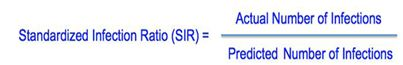Search Department of Public Health Filtered Topic Search

### What is the SIR, and What Does it Mean?

The Standardized Infection Ratio (SIR) is a statistic used to track healthcare associated infections (HAIs) over time, at a national, state, or facility level. The SIR compares the actual number of HAIs at each hospital, to the predicted number of infections. The predicted number is an estimate based on national baseline data, and it is risk adjusted. Risk adjustment takes into account that some hospitals treat sicker patients than others.

The formula for calculating the SIR is:What does the SIR tell me?

If the SIR is 1, then the number of actual infections is the same as the number of predicted infections.

If the SIR is less than 1, then the number of actual infections is less than the number of predicted infections.

If the SIR is greater than 1, then the number of actual infections is greater than the number of predicted infections.

Statistical Testing

Statistical tests are used to see if the difference between the number of actual infections, and the number of predicted infections are due to just chance alone. If it is extremely unlikely that the difference is due to chance, then the difference is called “statistically significant.”

If the SIR is less than 1, and the finding is statistically significant, then the facility’s performance is labeled Better than Expected.

If the SIR is greater than 1, and the finding is statistically significant, then the facility’s performance is labeled “Worse than Expected.

If the SIR is not statistically significant, then the facility's SIR is "In the expected range".

When the predicted number of infections is less than 1, then the “Numbers are too small to compare.

For more on the SIR, visit CDC’s website.

To contact the Healthcare Associated Infections Program, please call 860-509-7995.